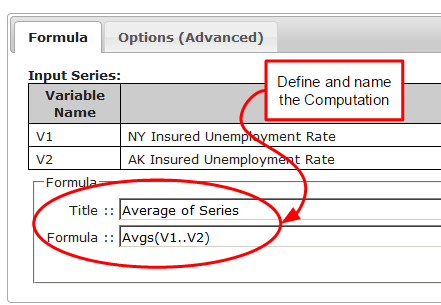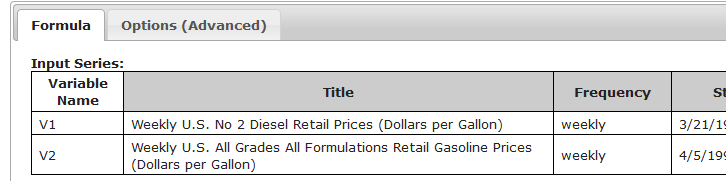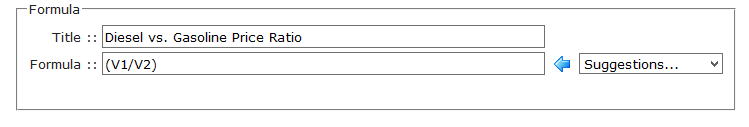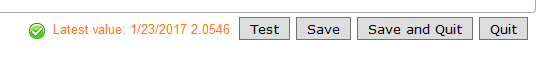# Calculations

Calculations are math formulas that derive new data series from other series.
 Immensely powerful,simple to use.• Calculations can use other calculated series as inputs
• All interdependencies are tracked and re-evaluated as needed
• Calculations are stored in your dataZoa account and evaluated in dataZoa's ComputeCloud
• A calculation in dataZoa works just like a cell in a spreadsheet, except that the "cell" contains an entire series!

## Creating

#### From the dropZone

From the dropZone, open the Gear menu, check Pick individual series, select your input series, then pop open Make a ComputeCloud Formula. See illustrations here.

#### From the Workbench

From the Workbench, checkbox the series you want to include, then click the + button in the header to pop open the formula editor. See illustrations here.

If you want to build several calculations from the same general group of data, consider using the dZCorkboard.

## Editing

To edit an existing calculation, use the dropZone or Workbench.

Your calculated series are marked with a blue formula flask. When you hover on the series and click on its flask
you get a menu with several calculation options. The first one opens the formula editor with the formula and setting for the calculation being edited.

### Tab: Formula

#### Inputs Section

 The Series you checked are the inputs to your Formula:#### Formula Section

 Use V1, V2, etc. as your formula variables:By their very nature, Formulas are an open-ended topic. These are excellent points of departure:

#### Buttons Section

 Use these buttons to test and finally save your Formula: